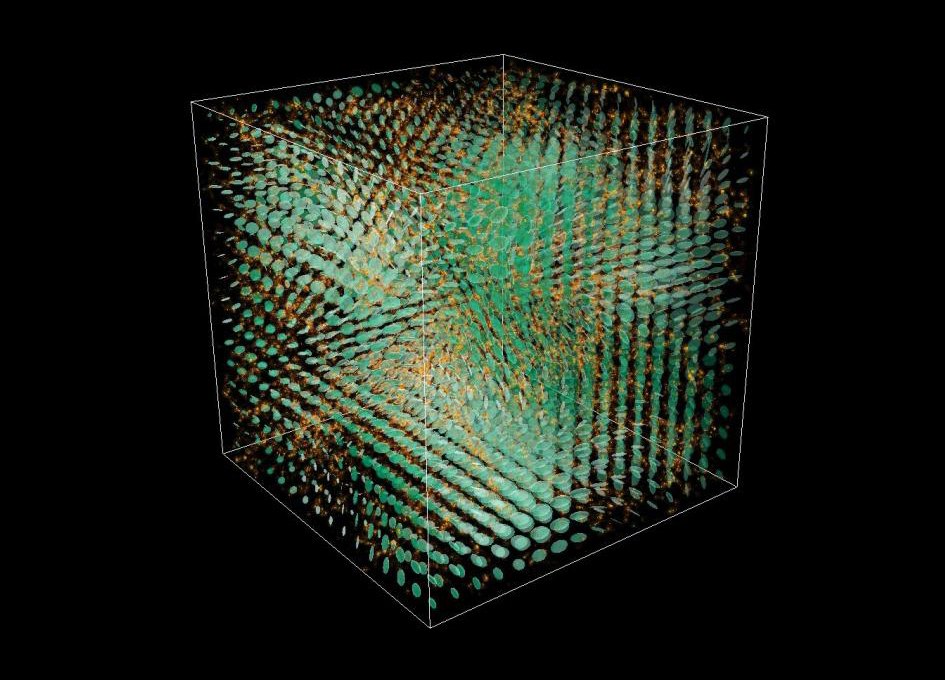Simulating The Expansion of the UniverseSImulation

The Universe is constantly expanding. It changes, creating new structures that merge. But how does our Universe evolve?

Physicists at the University of Geneva (UNIGE), Switzerland, have developed a new code of numerical simulations that offers a glimpse of the complex process of the formation of structures in the Universe. Based on Einstein's equations, they were able to integrate the rotation of space-time into their calculations and calculate the amplitude of gravitational waves, whose existence was confirmed for the first time on February 12, 2016. This study is published in the journal Nature Physics.

Until now, scientists studied the formation of large-scale cosmological structures based numerical simulations of Newtonian gravitation. These codes postulate that space itself does not change, it is said to be static, while time goes on. The simulations that it allows are very precise if the matter in the Universe moves slowly (i.e., about 300 km per second). However, when the matter particles move at high speed, this code only allows approximate calculations. Furthermore, it does not describe the fluctuations of dark energy. Constituting 70% of the total energy of the Universe (the remaining 30% is made of dark matter and ordinary matter), it is responsible for the accelerated expansion of the Universe. Therefore, it was necessary to find a new way to simulate the formation of cosmological structures and allow the study of these two phenomena.

The theory of general relativity applied

Ruth Durrer's team from the Department of Theoretical Physics in the Faculty of Science at UNIGE, has thus created a code, named gevolution, based on Einstein's Theory of general relativity. Indeed, general relativity considers space-time as being dynamical, that is to say that space and time are constantly changing, unlike the static space of Newtonian theory. The goal was to predict the amplitude and the impact of gravitational waves and frame-dragging (the rotation of space-time) induced by the formation of cosmological structures.

To do so, the physicists from UNIGE analysed a cubic portion in space, consisting of 60 billion zones with each containing a particle (that is to say, a portion of a galaxy), in order to study the way they move with respect to their neighbors. Thanks to the LATfield2 library (developed by David Daverio from UNIGE), which solves nonlinear partial differential equations, and the Supercomputer from the Swiss Supercomputer Center in Lugano, the researchers were able to study the motion of particles and calculate the metric (the measure of distances and time between two galaxies in the Universe) using Einstein's equations. The resulting spectra of these calculations allow to quantify the difference between the results obtained by gevolution and those coming from Newtonian codes. This allows to measure the effect of frame-dragging and gravitational waves introduced by the formation of structure in the Universe.

Gravitational waves and frame-dragging predicted by gevolution

Indeed, frame-dragging and gravitational waves have never been included in simulations until the creation of the gevolution code. This opens the way for the comparison of simulation results of the evolution of the Universe with observations. With their new code, the physicists at UNIGE will be able to test the theory of general relativity on much larger scales than at present. In order to open research to a maximum in this field, Professor Ruth Durrer and her team will make their gevolution code public. Perhaps soon light will be shed on the mysteries of dark energy.